# 一、单链表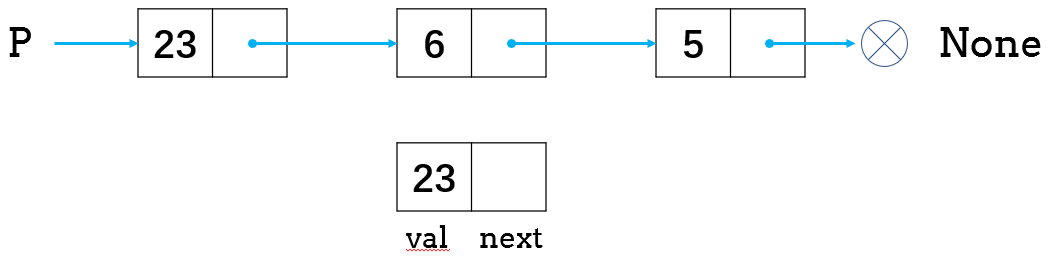## 1、插入新元素

• 初始化新节点cur

• curnext字段链接到prev的下个节点

• prev中的next字段链接到cur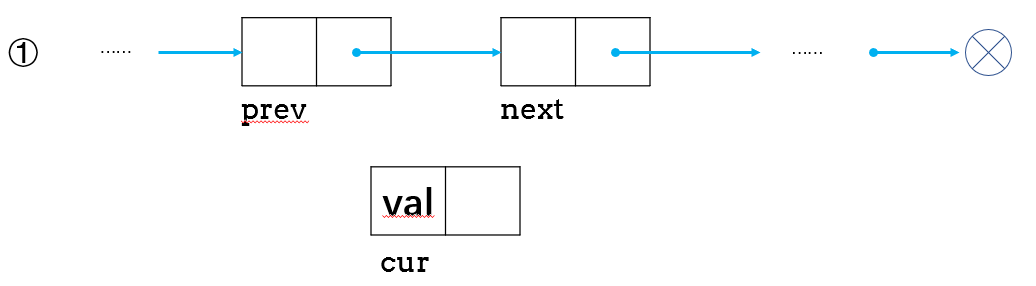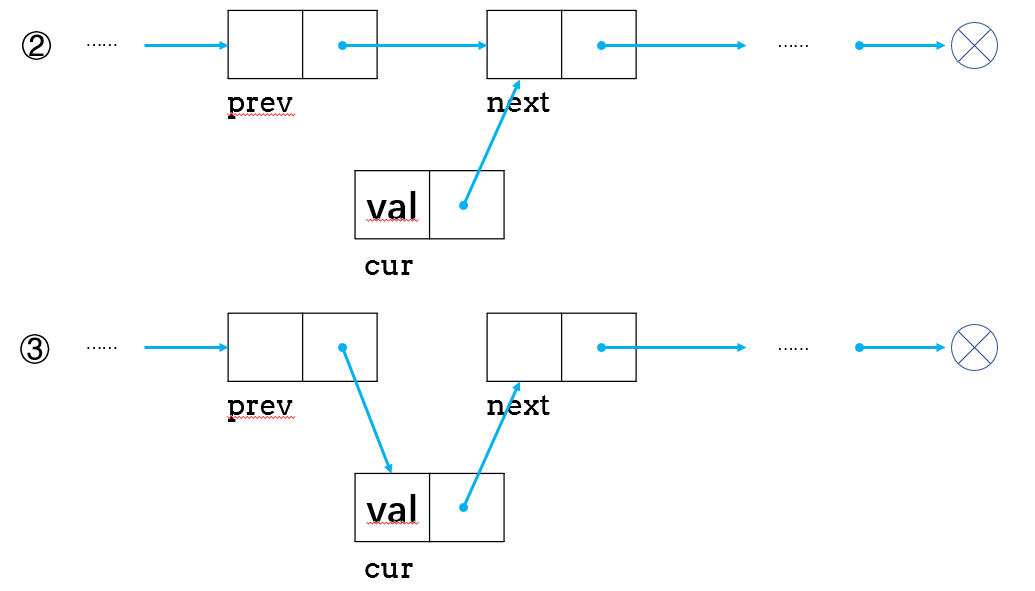## 2、在开头插入节点

• 初始化一个新节点cur

• 将新节点链接到原始头结点head

• cur指定为head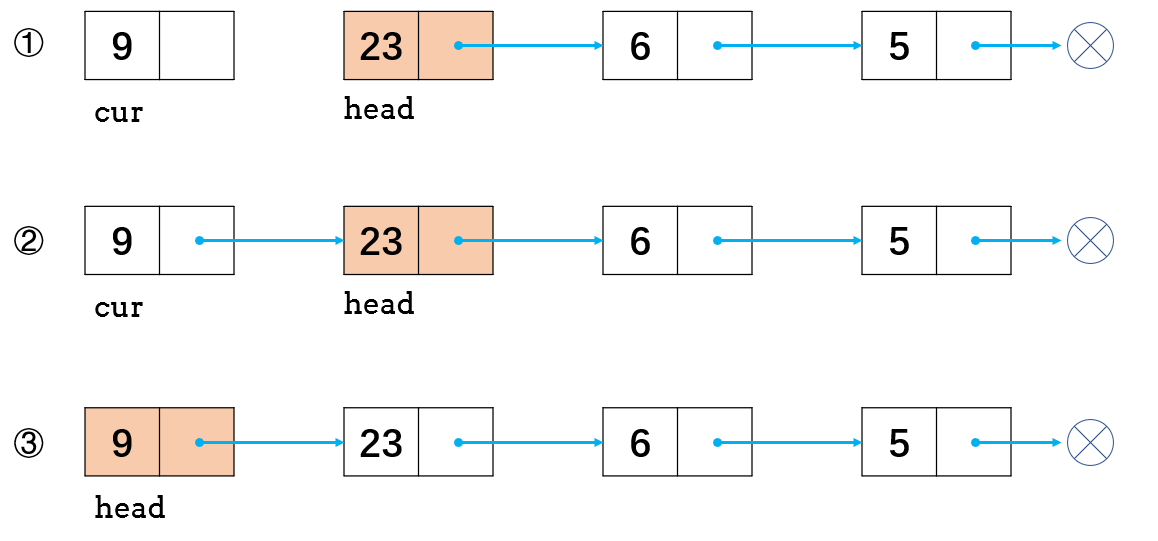## 3、删除操作

• 找到cur的上一个结点prev及其下一个结点next

• 链接prevcur的下一个结点next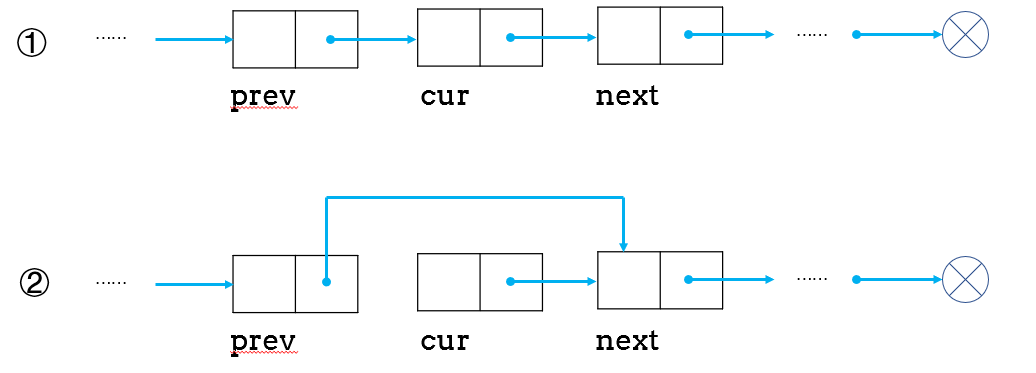## 4、删除第一个节点

• 给定原始链表和头结点head

• 将头结点的下个节点指定为头结点head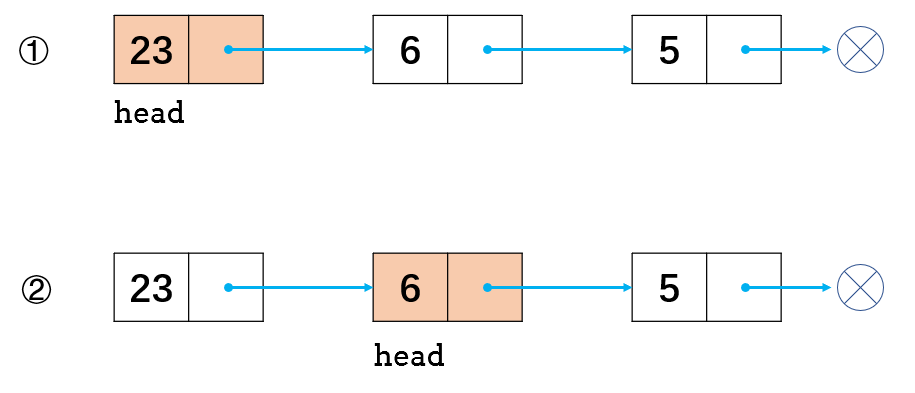## 5、单链表操作的复杂度

• 创建链表：$O(1)$
• 删除链表：在python里是$O(1)$
• 判断表空：$O(1)$
• 首端加入元素：$O(1)$
• 尾端加入元素，要找到表的最后节点：$O(n)$
• 定位插入元素：$O(n)$

• 首端删除元素：$O(1)$

• 尾端删除元素：$O(n)$
• 定位删除元素：$O(n)$
• 扫描、定位、遍历：$O(n)$

## 6、循环单链表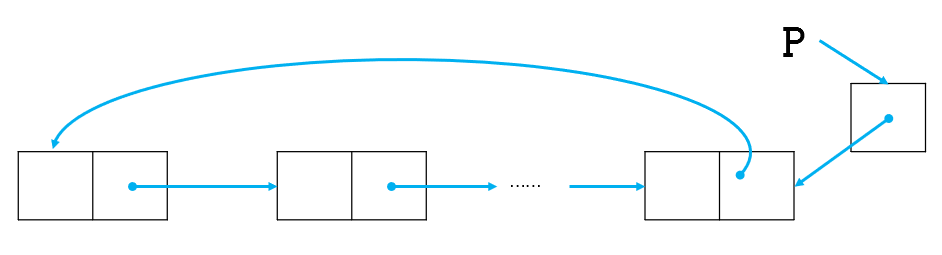# 二、双链表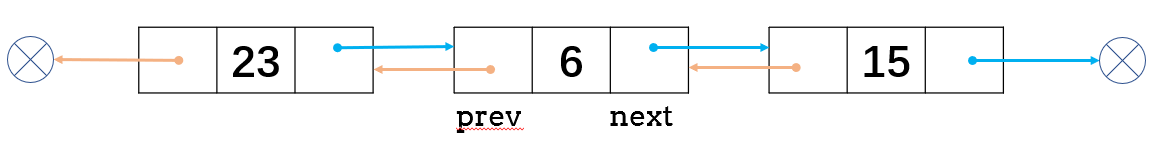## 1、添加操作

• 初始化一个新节点cur

• 链接curprevnext，链接curprevprev

• cur重新链接prevnext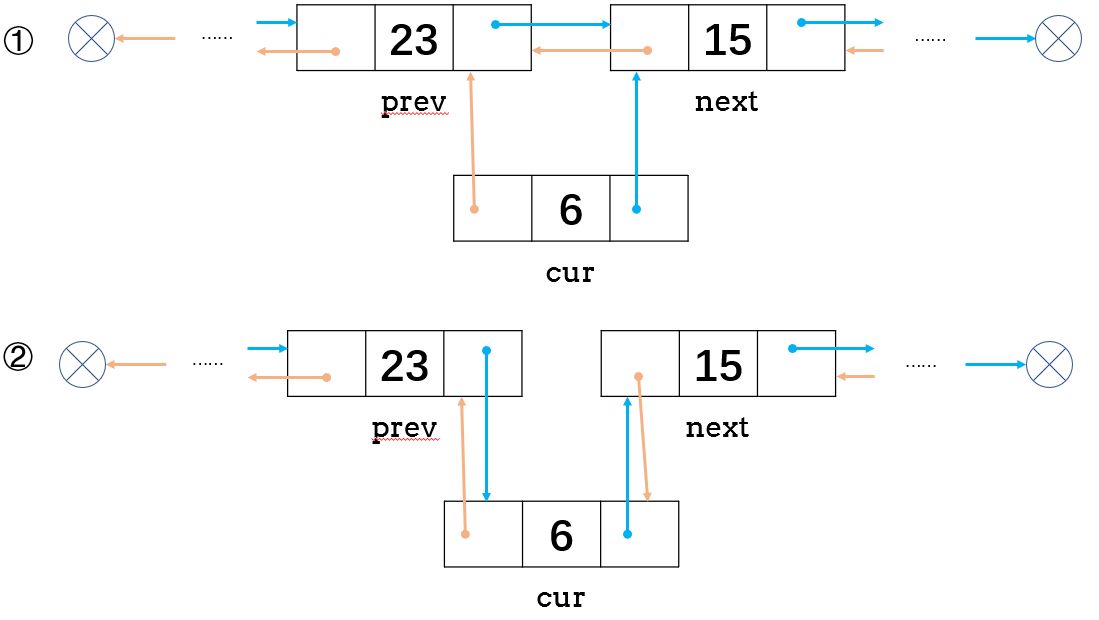## 2、删除操作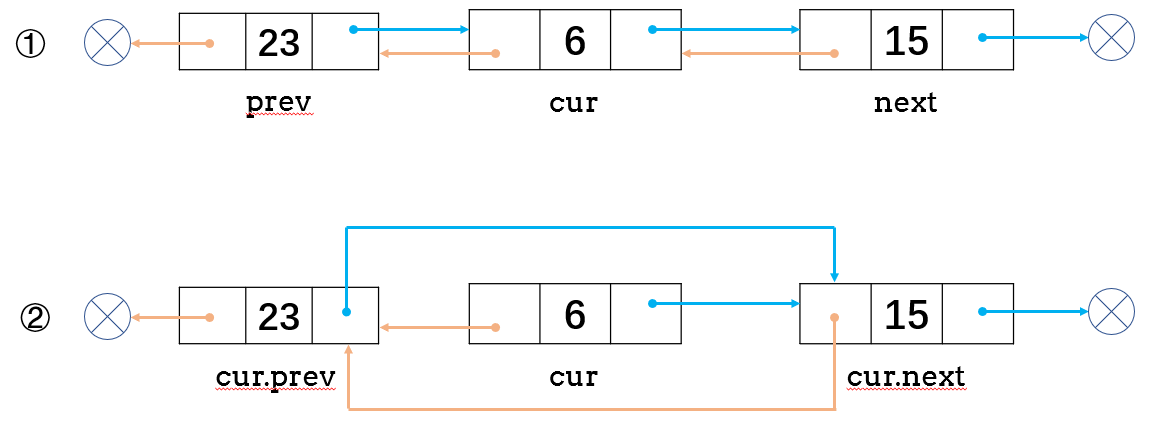## 3、循环双链表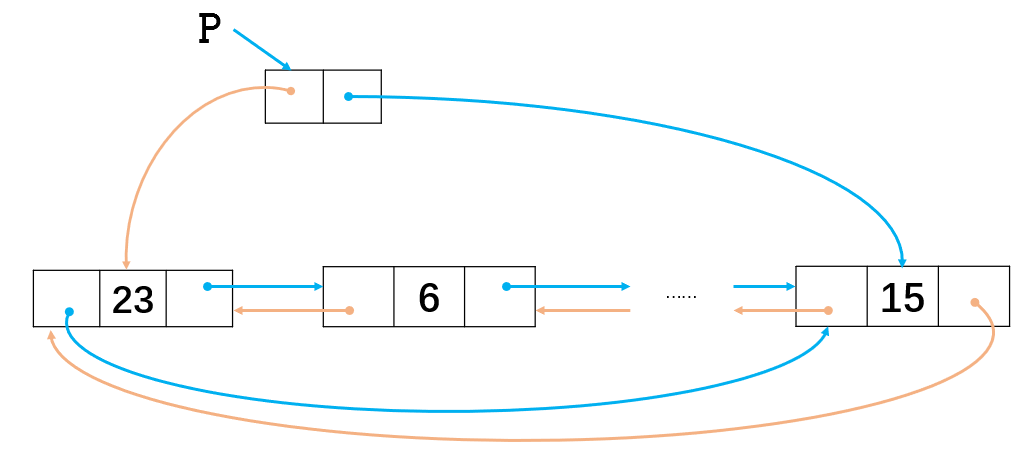# 三、代码实现

## 1、单链表

• get(index)：获取链表中第 index 个节点的值。如果索引无效，则返回-1

• addAtHead(val)：在链表的第一个元素之前添加一个值为 val 的节点。插入后，新节点将成为链表的第一个节点。

• addAtTail(val)：将值为 val 的节点追加到链表的最后一个元素。
• addAtIndex(index,val)：在链表中的第 index 个节点之前添加值为 val 的节点。如果 index 等于链表的长度，则该节点将附加到链表的末尾。如果 index 大于链表长度，则不会插入节点。
• deleteAtIndex(index)：如果索引 index 有效，则删除链表中的第 index 个节点。
# 节点类，代表链表的每个节点
class Node(object):
def __init__(self, x, next_=None):
# 节点的值
self.val = x
# 节点的下个指向
self.next = next_

# 链表类
# 初始化
def __init__(self):
# 初始化头部节点
# 记录链表长度
self.length = 0

# 打印链表所有节点
def print_all(self):
res = []
res.append(p.val)
while p.next:
p = p.next
res.append(p.val)
print(res)

# 根据索引index获得节点
def get(self, index):
"""
获得第index个节点
"""
# 头部节点
# 若链表为空或index小于0，则返回-1
if not p or index < 0:
return -1
# 遍历链表找到第index个节点
while index >= 1:
# 若index大于链表长度
if p.next == None:
return -1
else:
p = p.next
index -= 1
return p.val

"""
在头部添加节点
"""
self.length += 1

"""
在尾部添加节点
"""
# 如果链表为空，则在头部添加节点
self.length += 1
return
# 链表不为空，先获得头部节点
# 遍历节点，找到最后一个节点
while p.next is not None:
p = p.next
# 最后一个节点指向新建节点
p.next = Node(val)
self.length += 1

"""
在第index插入节点。若index等于链表长度，则在尾部添加，若大于节点长度，则节点不会被插入
"""
# 若index等于链表长度，节点添加为尾部
if index == self.length:
# 若index==0，节点添加在头部
elif index == 0:
# 若index大于链表长度或为负，不插入节点
elif index + 1 > self.length or index < 0:
return
else:
# 获得插入位置的前一个节点
while index > 1:
index -= 1
p = p.next
# 新建当前节点，next指向前一个节点的next
cur = Node(val, p.next)
# 前一个节点的next指向当前节点
p.next = cur
self.length += 1

def deleteAtIndex(self, index):
"""
删除第index个节点
"""
# 若index大于链表长度或为负，不删除节点
if index + 1 > self.length or index < 0:
return
# 若index==0，删除头部节点
elif index == 0:
# 重新指定头部节点为当前头部的next
self.length -= 1
return
else:
# 获得指定位置的前一个节点
while index > 1:
p = p.next
index -= 1
# 若前一个节点不为倒数第二个节点（也就是删除除头结点、尾节点以外的中间节点）
if p.next.next is not None:
p.next = p.next.next
# 若指定位置的前一个节点为倒数第二个节点（也就是删除尾节点）
else:
p.next = None
self.length -= 1

## 2、双指针应用

### （1）判断环形链表

输入：head = [3,2,0,-4], pos = 1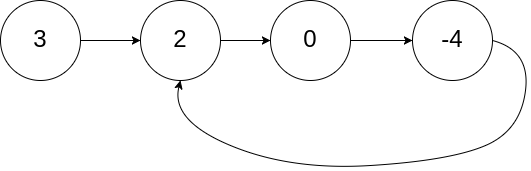输入：head = [1,2], pos = 0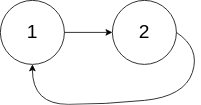输入：head = , pos = -1# Definition for singly-linked list.
# class ListNode(object):
#     def __init__(self, x):
#         self.val = x
#         self.next = None

class Solution(object):
"""
:rtype: bool
"""
'''
# 方法一 双链表
while p2 and p2.next:
p1 = p1.next
p2 = p2.next.next
if p1 == p2:
return True
return False
'''
### 方法二  哈希表
return False
hash_table=set()
return True
else:
return False

### （2）环形链表（二）

输入：head = [3,2,0,-4], pos = 1输入：head = [1,2], pos = 0输入：head = , pos = -1# Definition for singly-linked list.
# class ListNode(object):
#     def __init__(self, x):
#         self.val = x
#         self.next = None

class Solution(object):
"""
:rtype: ListNode
"""
while p2 and p2.next:
p1 = p1.next
p2 = p2.next.next
if p1 == p2:
while p != p1:
p = p.next
p1 = p1.next
return p
return None

### （3）相交链表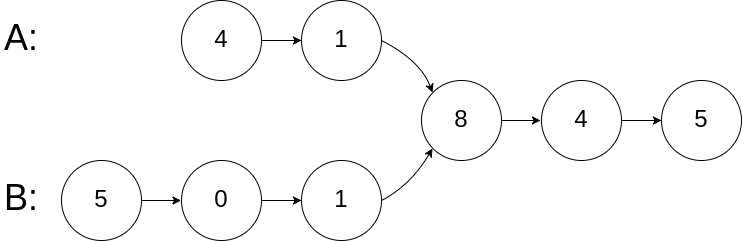输入：intersectVal = 8, listA = [4,1,8,4,5], listB = [5,0,1,8,4,5], skipA = 2, skipB = 3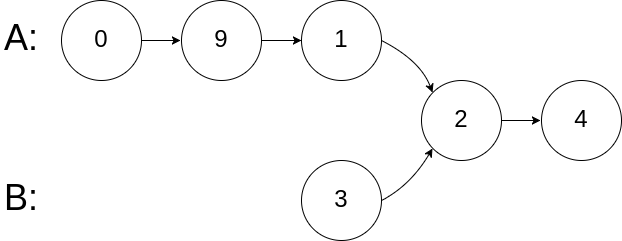输入：intersectVal = 2, listA = [0,9,1,2,4], listB = [3,2,4], skipA = 3, skipB = 1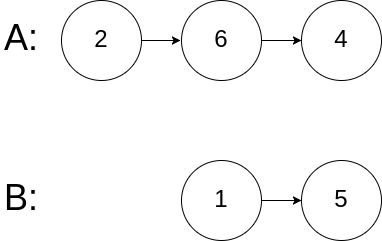输入：intersectVal = 0, listA = [2,6,4], listB = [1,5], skipA = 3, skipB = 2

• 如果两个链表没有交点，返回 null.
• 在返回结果后，两个链表仍须保持原有的结构。
• 可假定整个链表结构中没有循环。
• 程序尽量满足 O(n) 时间复杂度，且仅用 O(1) 内存。
# Definition for singly-linked list.
# class ListNode(object):
#     def __init__(self, x):
#         self.val = x
#         self.next = None

class Solution(object):
"""
:rtype: ListNode
"""
'''
# 方法一 哈希表
table = set()
while pa:
if pa not in table:
pa = pa.next
while pb:
if pb in table:
return pb
pb = pb.next
return None
'''
# 方法二 双指针
while pA != pB:
pA = pA.next if pA is not None else headB
pB = pB.next if pB is not None else headA
return pA

### （4）删除链表的导数第N个节点

给定一个链表: 1->2->3->4->5, 和 n = 2.

# Definition for singly-linked list.
# class ListNode(object):
#     def __init__(self, x):
#         self.val = x
#         self.next = None

class Solution(object):
"""
:type n: int
:rtype: ListNode
"""
'''
length = 0
table = []
while p:
table.append(p)
length += 1
p = p.next
if n == 1:
if length == 1:
return None
else:
table[-n-1].next = None
if n == length:
return table[-n+1]
table[-n-1].next = table[-n-1].next.next
'''
#构建双指针p1与p2，p1先走n步，然后一同运动，当p1指向表尾，p2指向的next即是倒数第N个节点，删除即可。
dummy = ListNode(0)

fast = slow = dummy
for i in range(n):
fast = fast.next
while fast.next:
fast = fast.next
slow = slow.next
slow.next = slow.next.next
return dummy.next